# F8Net只有8比特乘法的神经网络量化

### F8Net:只有8比特乘法的神经网络量化¶

【GaintPandaCV导语】

F8Net用定点化量化方法对DNN进行量化，在模型推理只有8-bit的乘法，没有16-bit/32-bit的乘法，采用非学习的方法即标准差来定小数位宽。目前是我看到的第一篇硬件层面全8-bit乘法的模型推理的方法。

##### 1.定点转换¶
以两个16位的浮点数相乘为例
2.918 × 3.1415926 = 9.1671672068

2.918 × 2^12 = 11952.168  定点化后取整为： 11952;
3.1415926× 2^12 = 12867.8632896 定点化后取整为： 12868;


###### 2.定点数相乘：¶
11952 * 12868 = 153798336.

###### 3.定点数还原为小数¶
153798336 / (2^24) = 9.167095184326171875



##### 问题2：为什么要做这样的量化，跟之前的量化有什么不同？¶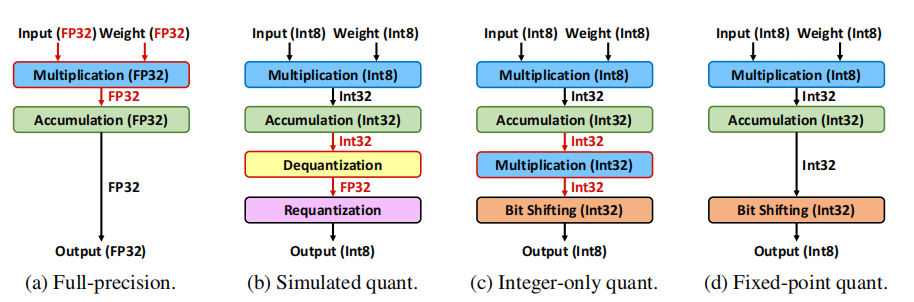1、模型量化推理只有8-bit位宽的乘法；
2、提出一个选择小数位宽的方法，对weight和activation都做定点化；
3、采用PACT的方法优化定点化的参数，把定点化和PACT的方法结合，推导出这样的优化公式；
4、定点化有效权重和有效偏差，有效权重和有效偏差指的是fold bn的con-bn的参数；
5、对残差块的d定点化参数对齐方法的实验和探究；
6、高精度的乘法对神经网络的性能来说，不是必须的。

##### 如何选择小数位宽：用标准差来选择小数位宽¶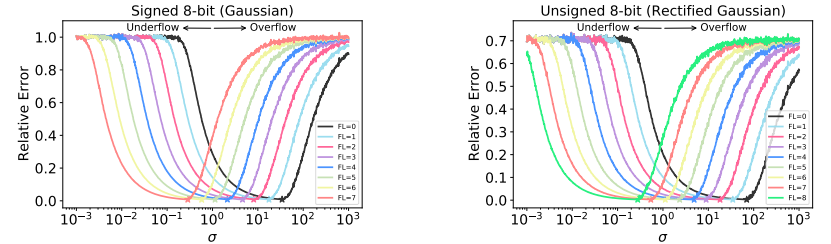ok，那么找到了一个选位宽的标准了，接下来呢，这个数值怎么计算呢？看另外一张图。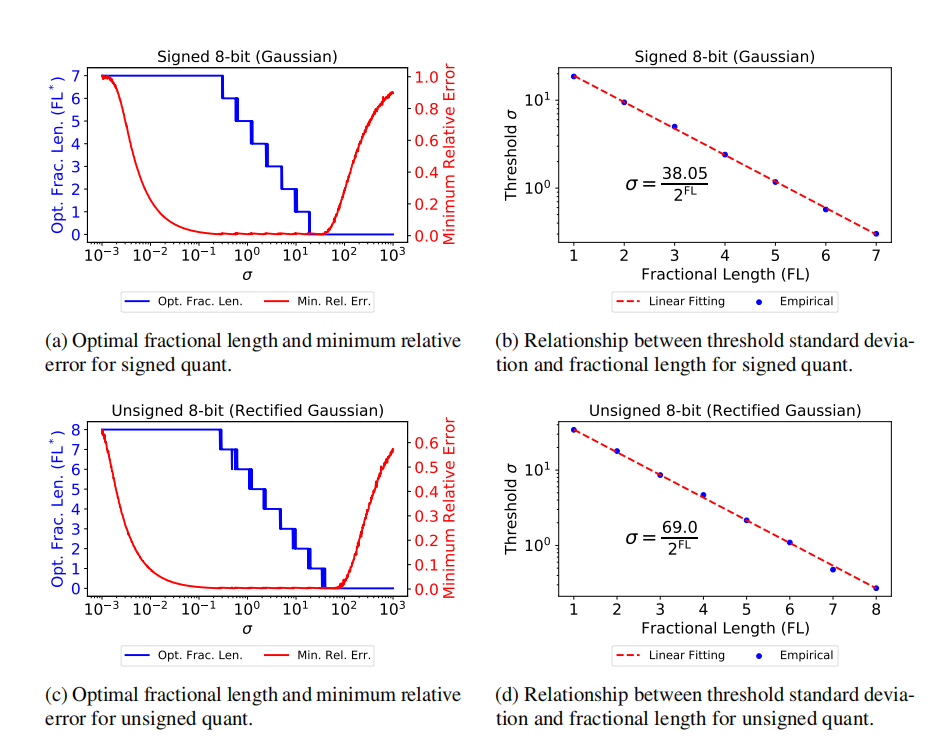##### 与PACT方法结合：找截断阈值¶

\operatorname{PACT}(x)=\frac{\alpha}{M} \operatorname{round}\left(\frac{M}{\alpha} \operatorname{clip}(x, 0, \alpha)\right) ,(1)
\text { fixquant }(x)=\frac{1}{2^{\mathrm{FL}}} \text { round }\left(\operatorname{clip}\left(x \cdot 2^{\mathrm{FL}}, 0,2^{\mathrm{WL}}-1\right)\right) ,(2)
\operatorname{PACT}(x)=\frac{2^{\mathrm{FL}} \alpha}{2^{\mathrm{WL}}-1} \text { fixquant }\left(\frac{2^{\mathrm{WL}}-1}{2^{\mathrm{FL}} \alpha} x\right), M=2^{\mathrm{WL}}-1 ,(3)
##### 有效权重weight与有效偏差bias¶

\begin{aligned} y_{b n} &=\frac{\gamma}{\sqrt{\sigma_{y}^{2}+\epsilon}}\left(\sum_{i}^{N} w_{i} x_{i}+b-\mu_{y}\right)+\beta \\ &=\gamma^{\prime}\left(\sum_{i}^{N} w_{i} x_{i}+b-\mu_{y}\right)+\beta \\ &=\sum_{i}^{N} \gamma^{\prime} w_{i} x_{i}+\gamma^{\prime}\left(b-\mu_{y}\right)+\beta \end{aligned} ,(4)

Fix scalling factor定义为:

\eta_{\mathrm{fix}}=\frac{2^{\mathrm{FL}} \alpha}{2^{\mathrm{WL}}-1} ,(5)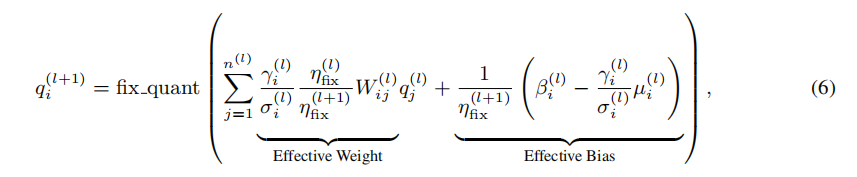##### 残差层的Fix scalling Factor¶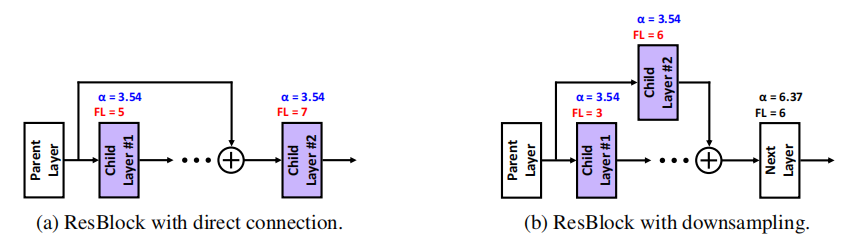OK，全文的方法都介绍完了。

##### 实验情况¶

1、传统的QAT：先训练一个全精度的模型；再加载这个pre-trained的模型参数，用完全相同的超参数来重新训练；使用F8Net的方法来选择小数位宽。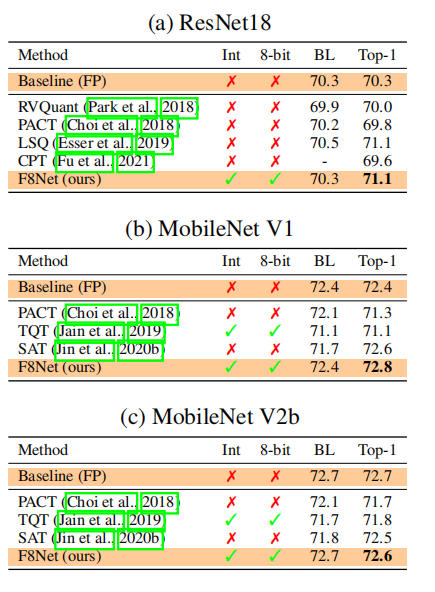2、Tiny fine-tuning的方法，来自于HAWQ_V3：加载一个pre-trained，保持很小的学习率(1e-4)，用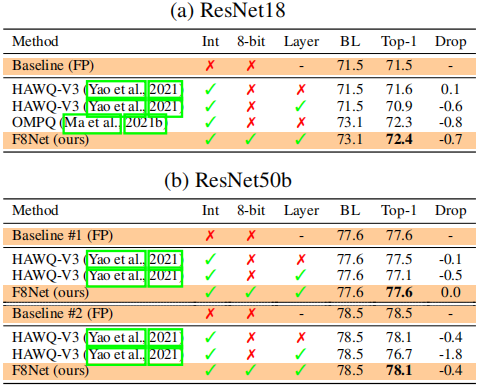128个数据训练500个iteration；使用网格搜索确定小数位宽。 在MobileNet的量化效果非常不错，基本可以达到无损量化。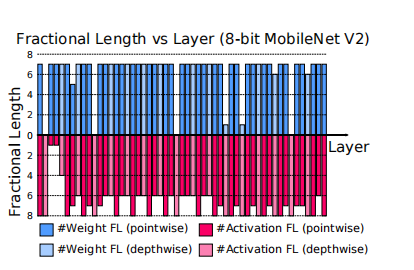##### 总结¶

F8Net首先是用IEEE 754标准定点化成8bit，用标准差来选择weight和activation的小数位宽，用PACT的方式来找截断阈值，把PACT的浮点类型的截断阈值融入有效weight和有效bias， 用有效weight和有效bias，对齐不同层的量化参数(这里指的是小数位宽和截断阈值)。个人觉得是个很优秀的工作，虽然一开始用标准差来定小数位宽，这第一眼看很简单，但认真一看，当中有很多细节需要解决。

Btw：看了openreview的“讨论”，我觉得这边工作还是很solid的。